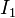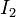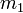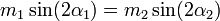# Blocks on two inclines of a free wedge

This article is about the following scenario. A freely movable triangular wedge placed on a fixed frictionless horizontal floor has two inclines$I_1$ and$I_2$ making angles$\alpha_1$ and$\alpha_2$ with the horizontal. There are blocks of masses$m_1$ and$m_2$, resting on the two inclines$I_1$ and$I_2$ respectively. All surfaces of contact are frictionless.$\! m_1 \sin(2\alpha_1) = m_2 \sin(2\alpha_2)$ The wedge remains stationary and the blocks both slide down their respective inclines.$\! m_1 \sin(2\alpha_1) > m_2 \sin(2\alpha_2)$ The horizontal push exerted by$m_1$ exceeds that by$m_2$, causing the wedge to move in the push direction of$m_1$. Both$m_1$ and$m_2$ slide down.$\! m_1 \sin(2\alpha_1) < m_2 \sin(2\alpha_2)$ The horizontal push exerted by$m_2$ exceeds that by$m_1$, causing the wedge to move in the push direction of$m_2$. Both$m_1$ and$m_2$ slide down.